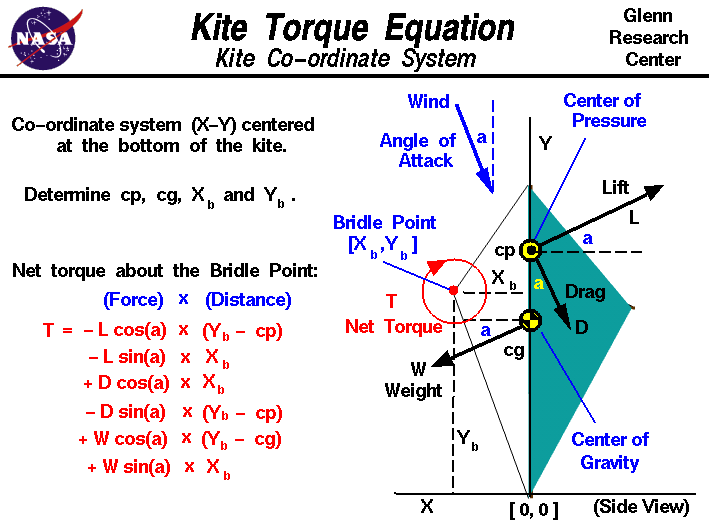+ Text Only Site
+ Non-Flash Version
+ Contact GlennNewton's first law of motion specifies that when all the external forces on an object are balanced, there is no net external force and the object moves at a constant velocity or remains at rest (velocity equals zero). This law holds for both linear motion and forces and for twisting motion and twisting forces. Twisting forces are called torques, or moments. The twisting motion occurs about some point called the pivot. A torque is related to a linear force; the torque about a point is equal to the force times the perpendicular distance to the point. In equilibrium, there is no net torque about the pivot and the angular velocity is constant (or zero). A kite flies because of the interactions of the forces on the kite. In order to fly, the aerodynamic lift, L, generated by the surface of the kite must be greater or equal to the weight, W, of the kite. The aerodynamic force which creates the lift also creates an aerodynamic drag, D, which is opposed by the tension in the control line. The kite is attached to the control line by a bridle string which is tied to the control line at the bridle point. The aerodynamic forces act through the center of pressure, cp, while the weight acts through the center of gravity, cg. In flight, the kite pivots about the bridle point because of the torques created by the weight and aerodynamic forces. On this page, we show the equation which describes the torque about the bridle point. To simplify the calculation, we choose a co-ordinate system X-Y which is centered at the base of the kite, with the Y-axis along the length of the kite, and the X-axis along the reference line for the computation of cg and cp. In this co-ordinate system, the wind approaches from above at the inclination angle, a, and the lift, drag, and weight vectors are all inclined at angle a to the co-ordinates. Knowing the location of cg, cp and the co-ordinates of the bridle point [xb,yb], we can compute the net torque T about the bridle point. Taking a positive torque as clockwise about the bridle point: T = - L * cos(a) * (yb - cp) - L * sin(a) * xb + D * cos(a) * xb - D * sin(a) * (yb - cp) + W * cos(a) * (yb - cg) + W * sin(a) * xb where sin(a) and cos(a) are the trigonometric sine and cosine of angle a. Notice that the distances from the bridle point are measured perpendicular and not just directly to the center of pressure and center of gravity. This produces two torque components for each force; the X-component of the force times the Y distance, and the Y-component of the force times the X distance. We can use the torque equation to determine the inclination angle a for which the torque T is zero. This is called the trim angle of the kite. As the kite rotates about the bridle point, the inclination angle between the kite and the wind changes. Since the magnitude and direction of the aerodynamic force depends on this angle, the aerodynamic torque about the bridle point also changes. When the aerodynamic torque balances the weight torque, the kite reaches an equilibrium, or balanced, condition and sits at a fixed inclination angle with no further rotation about the bridle point. But if the aerodynamic torque does not equal the weight torque, the kite continues to rotate under the action of the unequal torques. It is possible that the aerodynamic torque may never equal the weight torque for a given design and such a design would be called unbalanced. Considering a balanced design, the lift of the kite depends on the trim angle which is a constant. Since the weight of the kite is constant, the difference between the lift and the weight is also constant. If the lift minus weight is large, the kite will climb faster, fly higher, and is able to lift more string than if the lift minus weight is small. Since the trim angle depends on the balance of torques, and the torques depend on the location of the bridle point relative to the cg and cp, the location of the bridle point has a major effect on the performance of the kite. The location of the bridle point is set by the flyer before launch by moving the knot along the bridle string. If a kite naturally returns to an equilibrium condition when it is perturbed, the kite is said to be stable. To determine the kite stability we also use the torque equation given above. The mathematical equations involved with the forces and torques on a kite can be solved by using a computer program. You can use the KiteModeler program to further study how kites work and to design your own kites. Activities: Guided Tours Navigation ..Beginner's Guide Home Page+ Inspector General Hotline + Equal Employment Opportunity Data Posted Pursuant to the No Fear Act + Budgets, Strategic Plans and Accountability Reports + Freedom of Information Act + The President's Management Agenda + NASA Privacy Statement, Disclaimer, and Accessibility CertificationEditor: Nancy Hall NASA Official: Nancy Hall Last Updated: May 13 2021 + Contact Glenn